### Conjugate distributions¶

In :
import numpy as np
from numpy.random import beta
import matplotlib.pyplot as plt


#### Beta-Bernoulli¶

Let's look at the shape of the Beta distribution

In :
a, b = 1, 1
densities = plt.hist([beta(a,b) for _ in range(5000)], bins=10)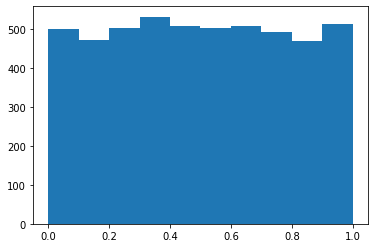Suppose we have observed a sequence of $n$ coin flips with $x$. Then the posterior -- what we know believe about $p$, given our prior and the new evidence -- also follows a Beta distribution.

Here is the derivation (from the Wiki page), where $s$ denotes "successes" (heads, here) and $f$ failures (so $n-x$).This is a Beta distribution with parameters $\alpha + s, \beta + f$.

In :
def posterior(a, b, x, n):
samples = [beta(a + x, b + n - x) for _ in range(10000)]
densities = plt.hist(samples, bins=10)
return np.array(samples)

In :
samples = posterior(.01, 1, 1, 1)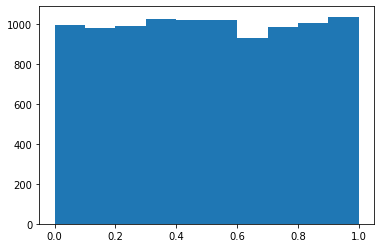In :
np.mean(samples)

Out:
0.6677448353537242
In :
samples = posterior_under_prior(1, .01, 1, 2)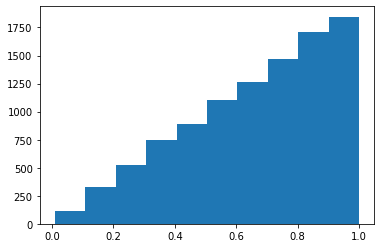In :
samples = posterior_under_prior(.01, 1, 1, 2)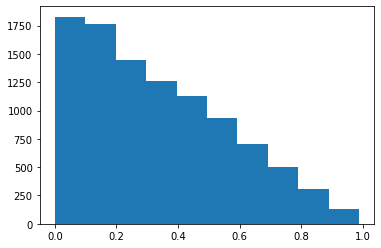In :
samples = posterior_under_prior(1, .001, 100, 200)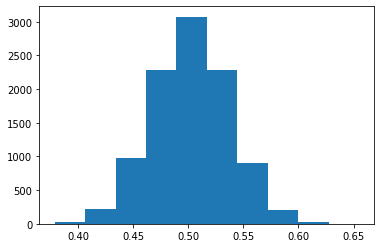#### Normal-Normal¶

There is a nice interactive demo here: https://stephens999.github.io/fiveMinuteStats/shiny_normal_example.html

In [ ]: